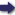## Bīrūnī, Muḥammad ibn Aḥmad, Alberuni's India (v. 2)

(London :  Kegan Paul, Trench, Trübner & Co.,  1910.)

 Tools

## Table of ContentsPrev Page 74 Next``` 74 ALBERUNTS INDIA. of its orbit, which is found in the computations of the corrections of sun and moon. AB is the diameter of the body of the sun, CD is the diameter of the earth, ODH is the cone of the shadow, HL is its elevation. Further, draw CE parallel to DB. Then is AE the difference between AB and OD, and the normal line CT is the middle distance of the sun, i.e. the radius of its orbit derived from the yojctnas of heaven (v. p. 72). From this the true distance of the sun always differs, sometimes being larger, sometimes smaller. We draw CK, which is of course determined by the parts of the sine. It stands in the same relation to CT, this being the sinus totits ( = radius), as the yojctnas of CK to the yojanas of OT. Hereby the measure of the diameter is reduced to yojanas. The yojanas of AB stand in the same relation to the yojancts of TO as the minutes of AB to the minutes of TO, the latter being the sinus totits. Thereby AB becomes known and determined by the minutes of the sphere, because the sinus totus is determined by the Quotations measuro of the circumference. For this reason Pulisa Brahma- '' says : " Multiply the yojctncts of the radius of the sphere Baiabiiadra. of the sun ov the moon by the true distance, and divide the product by the sinus totits. By the quotient you get for the sun, divide 22,278,240, and by the quotient you get for the moon, divide 1,650,240. The quotient then represents the minutes of the diameter of the body of either sun or moon." The last-mentioned two numbers are products of the multiplication of the yojanas of the diameters of sun and moon by 3438, which is the number of the minutes of the sinits totits. Likewise Brahmagupta says : " Multiply the yojanas of sun or moon by 3416, i.e. the minutes of the sinus totus, and divide the product by the yojctnas of the radius of the sphere of sun or moon." But the latter rule of division is not correct, because, according to it. ```Prev Page 74 Next